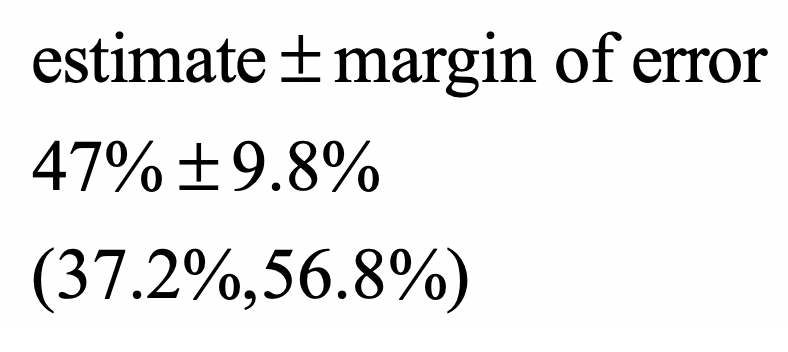Search

# What is Margin of Error?

Updated: Jan 10

Let's develop this concept with a very simple example: choosing a random sample of 100 students from East Kentwood High School (EKHS) and asking them whether or not they are taking an AP class. The population is all EKHS students and the true percent of students taking an AP class is 50%, but let's suppose we don't know this. We will use the percent from our sample as an estimate for the true percent of all EKHS students.

We take a random sample of 100 students. Are we guaranteed to get exactly 50 that are taking an AP class?

Of course not! It certainly would not be surprising to get 47 AP students or 52 AP students in our sample. It would even be possible for us to get 70 AP students (although very unlikely). Here is a dotplot of the results when we simulated this many times.We definitely see that 50% did occur in some of the simulations, but so did a bunch of other values, ranging from 40% to 62%. So here is the key takeaway:

Every time we take a random sample, we get a slightly different estimate for the true proportion of students taking an AP class. The above distribution of possible estimates is called a simulated sampling distribution and the fact that estimates vary from sample to sample is called sampling variability.

Because we know that estimates will vary from sample to sample, it doesn't make good sense for us to give our single estimate as what we think is the true percent for all EKHS students. Instead we calculate a 95% percent confidence interval by adding a subtracting a margin of error to our estimate. For example, let's suppose our sample of 100 students had 47 AP students:The reason we create an interval using the margin of error is because we know that each sample is going to give slightly different estimates. In other words, margin of error is our wiggle room to account for sampling variability.

Here the "error" is referring to the expected difference between our estimate and the truth that is the result of sampling variability.

## What Margin of Error is NOT

Margin of error is wiggle room to account for sampling variability. Here is a list of issues that margin of error does NOT account for:

Undercoverage: Let's suppose the sample of 100 EKHS students was taken from the students in the library after school on a Tuesday. Because students often stay after school to work on homework from AP classes, the estimate from this sample would be higher than the true percent of all EKHS students taking an AP class.

Nonresponse: Let's suppose the sample was taken through a survey that was emailed to 100 students, with only 60 students responding to the survey. Because AP students are more likely to respond to a school survey, the estimate from this sample would be higher than the true percent of all EKHS students taking an AP class.

Voluntary Response: Let's suppose the principal makes an announcement to the whole school that students should go to a website to fill out a school survey. Because AP students are more likely to respond to a school survey, the estimate from this sample would be higher than the true percent of all EKHS students taking an AP class.

Response Bias: Let's suppose the sample was done by having the principal interview each of the selected students. Because students might lie (in order to look good) and say they are taking an AP class when they are not, the estimate from this sample would be higher than the true percent of all EKHS students taking an AP class.

The margin of error that we use to calculate a confidence interval does not account for any of these issues.

Here, the "errors" are all mistakes that were made in designing the sampling method.

## What is an Error?

From the Marriam-Webster Dictionary:

the difference between an observed or calculated value and a true value

From the Cambridge English Dictionary:

something done by accident that is not correct, not accurate, or does not give the right result:

Here is the problem! The first definition jives with our definition of margin of error and the second one does not!

What the margin of error IS.

the difference between an observed or calculated value and a true value (due to sampling variability)

What the margin of error IS NOT.

something done by accident that is not correct, not accurate, or does not give the right result:

There is a simple solution to this problem.